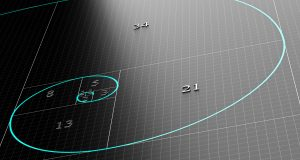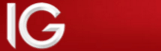## Fibonacci Retracement Indicators: Part I

### A little history about FibonacciTo understand Fibonacci Retracement Indicators, it is first necessary to understand where the numbers used for this indicator come from. All Fibonacci indicators use the same set of numbers that follow a simple mathematical sequence.

Fibonacci was the nickname of an Italian mathematician from the medieval ages; his real name is not entirely clear. Some documents refer to him as Leonardo Pisano, while in others, it appears his name was Leonardo Bigollo. This mathematician from the 12th century was already famous back in his times; he had been hired by no less than Fredrick II, the Holy Roman Emperor. He is attributed with having introduced Hindu-Arabic numerology based on the decimal system. However, nowadays he is probably better known for the Fibonacci sequence of numbers.

Fibonacci numbers and ratios

Leonardo Pisano, observed nature with particular detail, what he found led him to lay the foundations for a sequence of numbers that are repeated throughout nature. These numbers are often found to be the number of petals in a flower, the number of seeds in a sunflower or the number of segments in a pine cone.

It’s also important to understand that Fibonacci did not invent the indicators, but analysts have found that using Fibonacci numbers within these indicators makes them more powerful.

The sequence of numbers is also known as the Fibonacci Spiral, due to the graphical representation of the sequence. The graphical representation basically shows how the sequence grows in an exponential way.

## Use of the Fibonacci sequence in trading

As Fibonacci numbers are very frequent in nature, this repetitiveness of Fibonacci numbers must mean some connection between nature and statistics. It, therefore, seems intuitive to adopt these numbers when attempting to forecast price action and future resistance or support levels.

The sequence of numbers is created by adding the previous two numbers to obtain the next number in the sequence. So, starting with 0 and 1 the next number is 1, then you add 1 and 1, and the next number is 2. Continuing in this fashion the following numbers are 3, 5, 8, 13, 21 and so on. The series can be infinite but what these numbers are actually used for is to create ratios.

The ratios are used to establish how far a retracement in price could go; this helps traders in finding good entry points for the main trend. The ratios are calculated by taking a number and dividing it by the next higher number, by the 2nd higher number and by the 3rd higher number.

Division by the next higher number will give a ratio that tends to 0.618, or 61.8%. I say tends, as 5 divided by 8 equals 0.625, whereas, 89 divided by 144 equals 0.618056. The higher the numbers used in the sequence the closer the ratio will reach 0.618.

Dividing by the second higher number tends to the ratio of 0.382 or 38.2%, notice that 1-0.618 equals 0.382. The last ratio tends to 0.236; these ratios are then used to create a series of retracement and support levels. Repetition shows that often price will retrace from a high or low by approximately 61%, 38% or 23%. Also worthy of note is the fact that dividing a number by a preceding lower number the ratio obtained tends to 1.618. This number was known in ancient civilizations as the Golden Mean and often used in architecture.

These ratios may not mean much by themselves, but we will see later how they crop up on a regular basis as support and resistance levels. Read on here, How to apply fibonacci numbers to trading.Your capital is at risk Payout: 96% Min Deposit: £100 Bonus: None Regulated: YesYour capital is at risk